Related Tags

python
communitycreator

# How to implement Fourier Series in Python

Bindu Sanjana T

## Contents

• Fourier Series (Introduction, Definition, Key terms)
• Application of Fourier Series
• Fourier Series Expansion
• Implementing continuous wave functions using Python

## Fourier Series

The general concern when it comes to mathematical physics and engineering mathematics is to transform equations into a coordinate system where the expressions analyze, unravel, and are tractable to computation and analysis. The infrastructure to solve this problem of coordinate transformation was introduced by J.B Joseph Fourier in the early 18th century to investigate the theory of heat where he put forward the concept of the Fourier Series.

Fourier Series is a way of approximating arbitrary function (f(x)) as an infinite sum of sines and cosines of increasingly high frequency that provide an orthogonal basis for the space of solution functions. The sine and cosine functions present as eigenfunctions of the heat equation. The specific frequencies provided present as eigenvalues that are done according to the geometry with the boundary conditions determining the amplitudes.

You will come across the terms Fourier Series and Fourier Transform frequently. The difference between them is that the Fourier series is an expansion of periodic signal as a linear combination of sines and cosines, while Fourier transform is the process or function used to convert signals from the time domain to frequency domain.

To vividly understand how Fourier Series works the way we use in complex situations, one must also understand the advanced topics of Heat equations, Fourier Series, Complex Fourier Series, Discrete Fourier Transform (DFT), Fast Fourier Transform (FFT), Quantum Fourier Transform, Fourier Transform Spectroscopy, etc. In this shot, we will only go through the basics to understand what Fourier Series is and how it is used.

## Application of Fourier Series

1. The concepts of Hilbert spaces and operator theory are given mathematical foundation with the introduction of the Fourier Series concept.
2. It leads to successive revolution in the advancement of analytical and computational mathematics, data analysis, and numerical physics at an engineering scale.
3. Fast Fourier Transform (FFT) has enabled real-time image and audio compression, global communication networks.

In summary, the FFT has a more substantial and noteworthy role in shaping the modern world than any algorithm to date.

## Fourier Series expansion

The Fourier Series function (f(x)) can be represented as a periodic function. Any function is periodic with period L if it exhibits the same pattern after interval L along the X-axis.

The approximation of Fourier Series function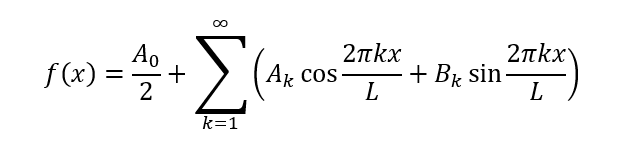This is called the Fourier Series expansion of a function. Where $A_{0}$, $A_{k}$, $B_{k}$ are called Fourier Coefficients.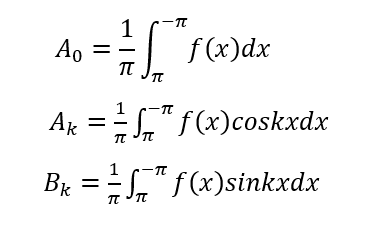$A_{0}$ represents the area under function f(x) in one time period (2*Pi) and is scaled by time period, it represents the average value. $A_{k}$ and $B_{k}$ represent the functions projected onto the particular cosine and sine wave.

Where, if,

• f(x) is even, i.e., f(-x)=-f(x) then $B_{k}$s=0

• f(x) is odd, i.e., f(-x)=f(x) then $A_{k}$s=0

For odd function with period 2L: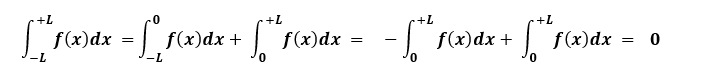For even function with Period 2L: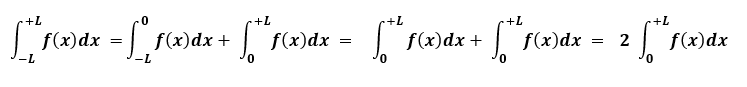## Implementing continuous wave functions in Fourier Series using Python:

Basically, what we aim to achieve while using the Fourier Series is to be able to draw the desired drawing by adding up a lot of waves with different oscillations (or) harmonic motions determined by periodic functions. There are basic waves that can be implemented and used to make other waves. The basic waves are:

• Square waves
• Sawtooth waves
• Triangular waves
• Semicircle waves

In, this shot we will focus on Square waves.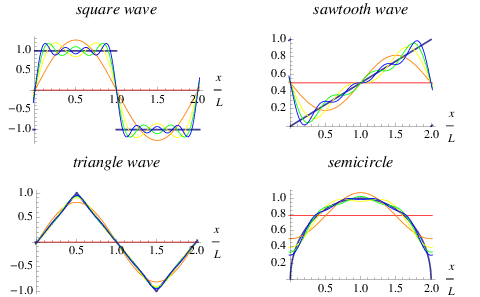In Python, we can make use of:

• SciPy.Signal module - to access Built-in piece wise continuous functions [square, sawtooth, etc.]
• SciPy.Integrate module - use quad for integration.
• math module - to use math for mathematical functions [sine, cosine, etc.]
• numPy module - to use lambda for defining functions.

Python code for generating a square wave:

import numpy as np

import matplotlib.pyplot as plt

from scipy.signal import square

from math import* //import all function from math

x=np.arange(-np.pi,np.pi,0.001) //x axis has been chosen from –π to +π, value
//of 1 smallest square along x axis is 0.001

y=square(x) //defining square wave function 𝑦 =−1, 𝑓𝑜𝑟 − 𝜋 ≤ 𝑥 ≤ 0
//y= +1, 𝑓𝑜𝑟 0 ≤ 𝑥 ≤ 𝜋

//define fuction

fc=lambda x:square(x)*cos(i*x)  //i :dummy index

fs=lambda x:square(x)*sin(i*x)

n=50 //max value of I, not taken infinity, better result with high value

An=[] // defining array

Bn=[]

sum=0

for i in range(n):

An.append(an)

for i in range(n):

Bn.append(bn) //putting value in array Bn

for i in range(n):

if i==0.0:

sum=sum+An[i]/2

else:

sum=sum+(An[i]*np.cos(i*x)+Bn[i]*np.sin(i*x))

plt.plot(x,sum,'g')

plt.plot(x,y,'r--')

plt.title("fourier series for square wave")

plt.show()


The graph will look like: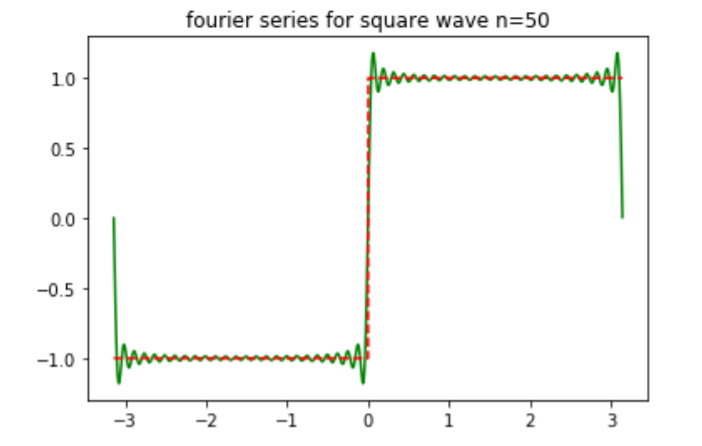Example: Square Wave

𝒇(𝒙) = {𝟎, −𝑳 ≤ 𝒙 ≤ 𝟎}

𝒇(𝒙) = {𝟏, 𝟎 ≤ 𝒙 ≤ 𝑳}

f(x) has period 2L

for L=0.5, Period=1

import matplotlib.pyplot as plt

from scipy.signal import square

L=1

x=np.arange(-L,L,0.001)

y=square(2*np.pi*x)

plt.plot(x,y,'r--')

plt.title("fourier series for square wave ")

plt.show()



The generated graph is: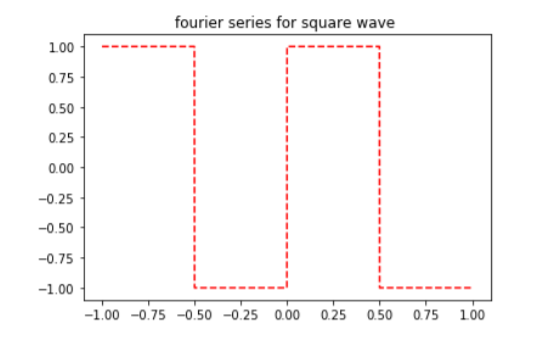RELATED TAGS

python
communitycreator

CONTRIBUTOR

Bindu Sanjana T
RELATED COURSES

View all Courses

Keep Exploring

Learn in-demand tech skills in half the time